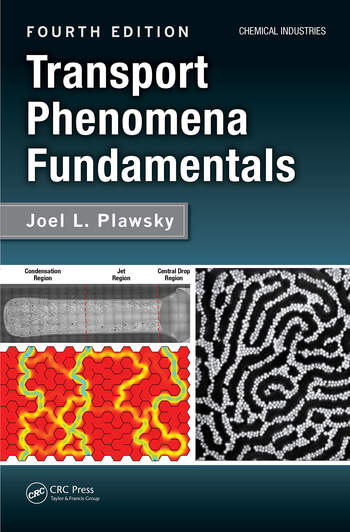# Transport Phenomena Fundamentals

## 4th Edition

Joel L. Plawsky

CRC Press
March 19, 2020 Forthcoming
Textbook - 840 Pages - 510 B/W Illustrations
ISBN 9781138080560 - CAT# K34480
Series: Chemical Industries

For Instructors Request Inspection Copy

was \$159.95

USD\$127.96

SAVE ~\$31.99

Available for pre-order. Item will ship after March 19, 2020
FREE Standard Shipping!

## Preview

### Summary

The fourth edition of Transport Phenomena Fundamentals continues with its streamlined approach to the subject, based on a unified treatment of heat, mass, and momentum transport using a balance equation approach. The new edition includes more worked examples within each chapter and adds confidence-building problems at the end of each chapter. Some numerical solutions are included in an appendix for students to check their comprehension of key concepts. Additional resources online include exercises that can be practiced using a wide range of software programs available for simulating engineering problems, such as, COMSOL®, Maple®, Fluent, Aspen, Mathematica, Python and MATLAB®, lecture notes, and past exams. This edition incorporates a wider range of problems to expand the utility of the text beyond chemical engineering.

The text is divided into two parts, which can be used for teaching a two-term course. Part I covers the balance equation in the context of diffusive transport—momentum, energy, mass, and charge. Each chapter adds a term to the balance equation, highlighting that term's effects on the physical behavior of the system and the underlying mathematical description. Chapters familiarize students with modeling and developing mathematical expressions based on the analysis of a control volume, the derivation of the governing differential equations, and the solution to those equations with appropriate boundary conditions.

Part II builds on the diffusive transport balance equation by introducing convective transport terms, focusing on partial, rather than ordinary, differential equations. The text describes paring down the full, microscopic equations governing the phenomena to simplify the models and develop engineering solutions, and it introduces macroscopic versions of the balance equations for use where the microscopic approach is either too difficult to solve or would yield much more information that is actually required. The text discusses the momentum, Bernoulli, energy, and species continuity equations, including a brief description of how these equations are applied to heat exchangers, continuous contactors, and chemical reactors. The book introduces the three fundamental transport coefficients: the friction factor, the heat transfer coefficient, and the mass transfer coefficient in the context of boundary layer theory. Laminar flow situations are treated first followed by a discussion of turbulence. The final chapter covers the basics of radiative heat transfer, including concepts such as blackbodies, graybodies, radiation shields, and enclosures.

#### Instructors

We provide complimentary e-inspection copies of primary textbooks to instructors considering our books for course adoption.